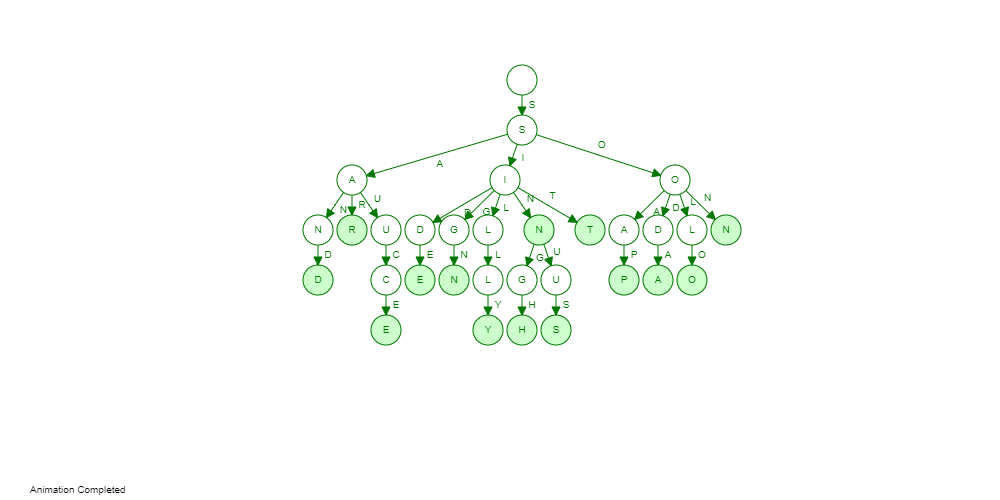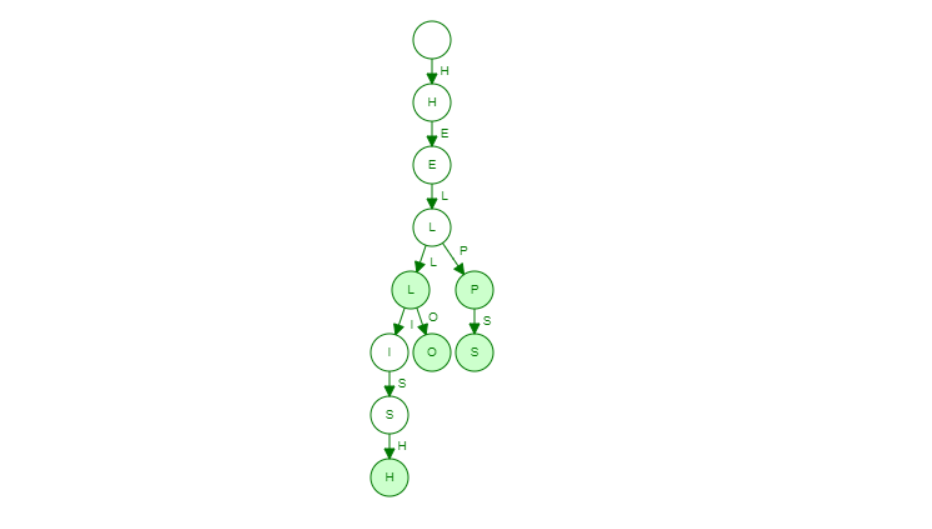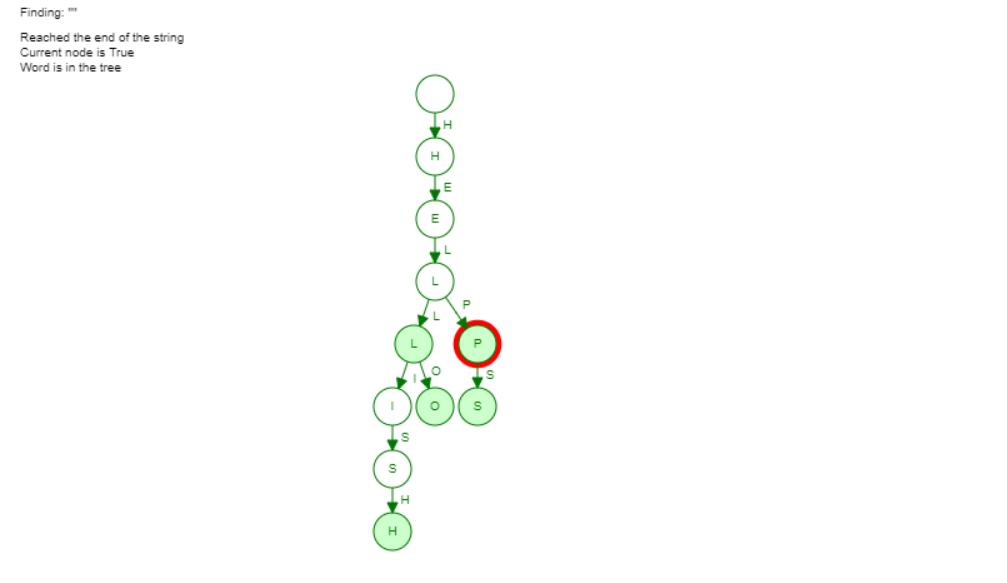×

Search anything:

# Autocomplete feature using TRIE Data Structure

#### Algorithms Data Structures Software EngineeringGet this book -> Problems on Array: For Interviews and Competitive Programming

Reading time: 35 minutes | Coding time: 10 minutes

Autocomplete is a feature of suggesting possible extensions to a partially written text and is widely used in search engine, code IDEs and much more. Trie data structure is a perfect fit to implement this feature efficient in terms of memory and time [O(length of string)]. In this article, we will explore this in depth and implement this as well.

Before going into the details, we will go through basic details of Trie as a data structure to understand the concept deeply.

Following is the autocomplete feature in Google Search Engine:TRIE Data Structure also known as the Prefix Tree or Radix Tree is remarked as an excellent Data Structure for processing and storing the Strings and is primarily used for word retrieval. The nodes in the TRIE Data Structure are used for storing the associate keys of strings and values that are being stored in the TRIE.

Autocomplete is one of the most popular features utilized in Search Engines from the User Experience (UI) perspective and to implement this feature, we will look at the working of TRIE Data Structure to implement a basic Autocomplete Feature.

What makes a TRIE Data Structure so optimized for performing such actions? Unlike the popular Binary Trees, each node in TRIE can contain a maximum of 26 nodes which are pointers to the 26 different alphabets of English Language. The position of each node in a TRIE defines the key with which the node is associated. This makes the TRIE indexing suitable for maintaining the variable sized key values. The actual key values is never stored but the key values are implied through links. A TRIE of Order 26 can maintain a whole English dictionary.We will be implementing a basic TRIE Data Structure for Autocomplete. The primary aim for our code will be for the program to return a list of words if it is given a simple query. Example: If given a query, "me", the TRIE should return {"meta","mega","methane"}. We will implement the code in Python-3 Programming Language which is a powerful General-Purpose Programming Language.

## Working of Autocomplete Feature on TRIE Data Structure

Here we will use a TRIE Data Structure Visualizer available here to visualize how words are inserted and how strings are found in a TRIE Data Structure. We will input a list of five words: ["Hell","Hello","Help","Helps","Hellish"]. Let's input them into our TRIE Data Structure:This is the implementation of our basic TRIE Data Structure. Next we will try to find a word "Help" in this TRIE Data Structure. It will jump from node to node and when the end of the leaf node approaches, it processes the string and displays the string."Help" is proccesed by starting up from the root node and comparing each node as it moves down. Here the traversal can be viewed as H->E->L->P as the search terminates due to the end of the string. If we have taken another word "Helping" then the traversals would look like H->E->L->P->EndOfWord . The string could not be found in the TRIE as the end of the string is reached and the search is terminated.

As we insert keys in the TRIE Data Structure, each input key is inserted as an individual node and perform the role of an index to further child nodes. If our input is a prefix of an existing string present in the TRIE, then we can mark the new node as a leaf node to the pre-defined key. Otherwise, the new keys are constructed and the last node is marked as the Leaf Node. Search Operation is relatively easy in TRIE as a Search Operation starts from the Root Node and moves ahead by comparing characters. If any character does not exist, then no such string is found in the TRIE Data Structure.

Here is the Pseudocode of inserting a string in a trie:

``````// Insertion of a Node

void insertionOfaString(String word)
{
for(Every Character in String word)
{
if(Child Node for Character is NULL)
{
Child_Node=new Node();
}
Current_Node=Child_Node;
}
}
``````

Following is the pseudocode for searching a node:

``````// Searching of a Node

boolean searchingOfaNode(String word)
{
for(Every Character in String word)
{
if(Child Node for Character is NULL)
{
return false;
}
}
return true;
}
``````

Our autocomplete function will be an extension of the search feature. Once we have found the string in our trie, we need to do a depth first search or breadth first search from that node (as a head node) and keep a list of all complete strings encountered in the process. This list of strings is our list of autocomplete words.

Following is the pseudocode of our autocomplete function:

``````# can be DFS or BFS
def traversal(self, item):
if self.leaf:
print (item)
for i in self.next:
s = item + i
self.next[i].traversal(s)

# actual autocomplete feature
def autocomplete(self, item):
i = 0
s = ''
while i < len(item):
k = item[i]
s += k
if k in self.next:
self = self.next[k]
else:
i += 1
self.traversal(s)
return 'END'
``````

Now we will see the Code Implementation of our TRIE Data Structure and how we can process an autocomplete query.

## Code Implementation

We will first create a Class trieNode which will consist of all the member functions that are required to implement the Autocomplete feature. We will also implement the Leaf Function which will be required to indicate the end of the string:

``````class trieNode:
def __init__(self):
self.next={}
self.leaf=False # Indicates no other Leaf Node
``````

As our trieNode class has been declared and our init Constructor (In Strict Object Oriented Principles) has been implemented to mark, the end of the string, we will now implement a function add_item() which can add a string to add a string to the TRIE.

``````def add_item(self,item):
i=0
while i < len(item):
k = item[i]
if not k in self.next:
node = trieNode()
self.next[k] = node
self = self.next[k]
#Leaf Node if Last Character
if i == len(item) - 1:
self.leaf = True
else:
self.leaf = False
i += 1
``````

Now we are going to implement a function search() which can search for a given string in our TRIE Data Structure which we hav initialized and created in our previous two functions.

``````def search(self, item):
if self.leaf and len(item) == 0:
return True
first = item[:1] #Gets First Character of String
str = item[1:]   #Gets Remaining Character of String
if first in self.next:
return self.next[first].search(str)
else:
return False
``````

We have implemented all the basic functions which can initialize a TRIE Data Structure, input all the strings from our collection and also search for a string. Now we will implement the primary functions to perform autocompletion. The function will traverse till the last of the passed query inside the TRIE Data Structure and can then perform a Traversal from the current node element to get all the Child Nodes which can then be further concatenated to get our final strings.

In the autocomplete() function, we will pass the query whose autocomplete search results needs to be processed and returned. We will implement a While Loop which would run throughout the entire length of our search query. We will extract the characters from the string passed to the function and will process it with the TRIE Data Structure that has been implement earlier. If the node associated with the extracted character exists, it is added and processed during the traversal and an "END" is returned to signify the end of the autocomplete results.

``````def traversal(self, item):
if self.leaf:
print (item)
for i in self.next:
s = item + i
self.next[i].traversal(s)

def autocomplete(self, item):
i = 0
s = ''
while i < len(item):
k = item[i]
s += k
if k in self.next:
self = self.next[k]
else:
i += 1
self.traversal(s)
return 'END'
``````

Next we will implement a Driver Function to check whether our Code is working fine or not. We will also define a list of various words which can be inputted to the TRIE Data Structure and a query which can be processed to give all the strings matching the Query.

``````list = [
'sin',
'singh',
'sign',
'sinus',
'sit',
'silly',
'side',
'son',
'soda',
'sauce',
'sand',
'soap',
'sar',
'solo',
'sour',
'sun',
'sure',
'an',
'ant',
'aunt',
'hell',
'hello',
'help',
'helps',
'hellish',
]
x = trieNode()
for i in list:
print (x.autocomplete('hel'))
``````

The Output of the Driver Function would be:

``````hello
hellish
helps
END
``````

Here is the complete source code of our implementation:

``````class trieNode:
def __init__(self):
self.next = {}
self.leaf = False
i = 0
while i < len(item):
k = item[i]
if not k in self.next:
node = trieNode()
self.next[k] = node
self = self.next[k]
if i == len(item) - 1:
self.leaf = True
else:
self.leaf = False
i += 1
def search(self, item):
if self.leaf and len(item) == 0:
return True
first = item[:1]
str = item[1:]
if first in self.next:
return self.next[first].search(str)
else:
return False
def traversal(self, item):
if self.leaf:
print (item)
for i in self.next:
s = item + i
self.next[i].traversal(s)
def autocomplete(self, item):
i = 0
s = ''
while i < len(item):
k = item[i]
s += k
if k in self.next:
self = self.next[k]
else:
i += 1
self.traversal(s)
return 'END'
list = [
'sin',
'singh',
'sign',
'sinus',
'sit',
'silly',
'side',
'son',
'soda',
'sauce',
'sand',
'soap',
'sar',
'solo',
'sour',
'sun',
'sure',
'an',
'ant',
'aunt',
'hell',
'hello',
'help',
'helps',
'hellish',
]
x = trieNode()
for i in list:
print (x.autocomplete('he'))
``````

The advantage of using TRIE Data Structure in implementation of Autocomplete Feature are briefly described value:

• No Collision of different Keys in a TRIE Data Structure make it an effective Data Structure for storing lexicographic words found in a Dictionary.
• The Best Case Time Complexity has been described as O(1) while the Average and Worst Case is described as O(Length-Of-Key).
• It can be optimized to support larger collection of words using Compact TRIE Data Structure Implementations.#### Harsh Bardhan Mishra

Intern at OpenGenus

Autocomplete feature using TRIE Data Structure